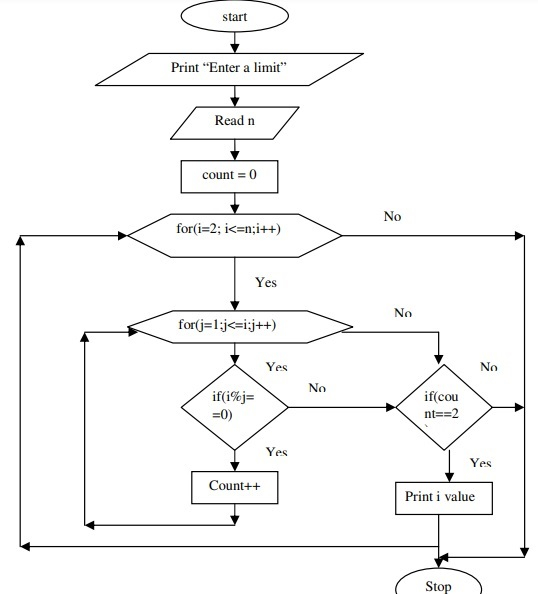# C program to display all prime numbers between 1 to N using for loop

## Problem

Write a C program to display all the prime numbers between 1 and n is a value given by the user at run time.

## Solution

C program to display all the prime numbers between 1 and n is a value given by the user at run time is explained below −

## Algorithm

Given below is an algorithm to display all the prime numbers between 1 and n is a value given by the user at run time.

Step 1 − Read n value.

Step 2 − Initialize count = 0

Step 3 − for i = 2 to n

   a. for j = 1 to i
b. if i % j = 0
c. then increment count
d. if count is equal to 2
e. then print i value

## Flowchart

A flowchart is given below to explain the algorithm for the C program to display all the prime numbers between 1 and n is a value given by the user at run time.## Example

Following is the C program to display all the prime numbers between 1 and n is a value given by the user at run time

Live Demo

#include<stdio.h>
void main(){
int i, num, n, count;
printf("Enter the range: ");
scanf("%d", &n);
printf("The prime numbers in between the range 1 to %d:",n);
for(num = 1;num<=n;num++){
count = 0;
for(i=2;i<=num/2;i++){
if(num%i==0){
count++;
break;
}
}
if(count==0 && num!= 1)
printf("%d ",num);
}
}

## Output

When the above program is executed, it produces the following result −

Enter the range:50
The prime numbers in between the range 1 to 50:
2 3 5 7 11 13 17 19 23 29 31 37 41 43 47